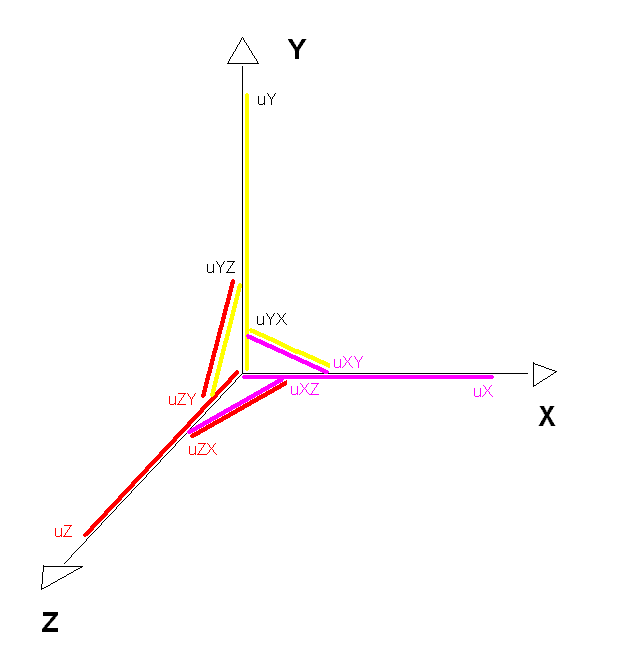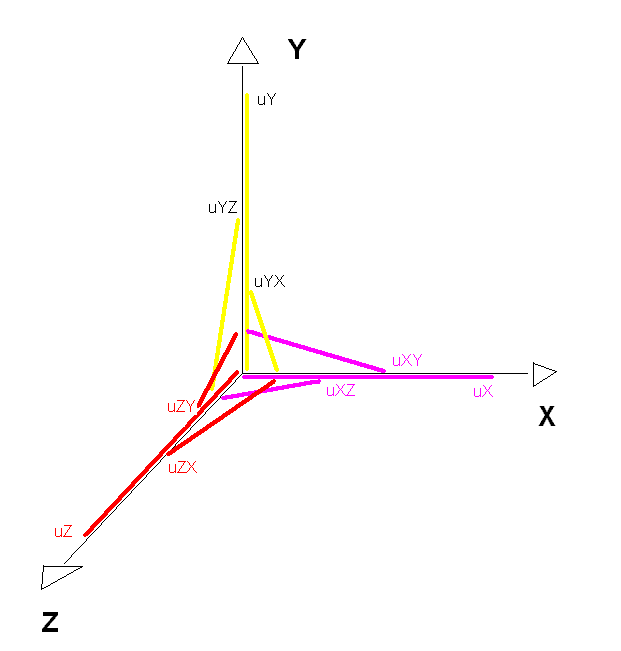# of Karim Belabas, Aïssa Derhem, and Daniel C. Mayer

 * On these pages, we present most recent results of our joint research, directly from the lab. * Basic bibliography: K. Belabas, A fast algorithm to compute cubic fields, Math. Comp. 66 (1997), 1213-1237 A. Derhem, Capitulation dans les extensions quadratiques de corps de nombres cubiques cycliques, Thèse de doctorat, Université Laval, Quebec, 1988 D. C. Mayer, Multiplicities of dihedral discriminants, Math. Comp. 58 (1992), 831-847 and S55-S58 * E-mail addresses: Karim.Belabas@math.u-psud.fr aderhem@yahoo.fr danielmayer@algebra.at *

 Voronoi Type Lattices in Cyclic Cubic Fields (2002/04/26) Dan (02/04/26): Using VORONOI's algorithm for signature (3,0), published in his doctoral dissertation, we can determine the geometry of lattice minima in the discrete geometric image of O_L, the maximal order of any cyclic cubic field L, racing along the VORONOI HighWays in various directions. Dan (02/04/26): First an example of inverse associations, where uYX = uXY^-1, uZY = uYZ^-1, uXZ = uZX^-1. This structure occurs for the conductors f = 61, 331, 547, ... Chain in X-direction: no pre period v = 0, chain length pl_X = 6, N(n_X^6(1)) = 1 X-Branch in Y-direction: branch length bl_XY = 1, association on X-chain at position ap = 2, N(n_Y^1(1)) = N(n_X^2(1)) X-Branch in Z-direction: branch length bl_XZ = 2, association on X-chain at position ap = 1, N(n_Z^2(1)) = N(n_X^1(1)) The symmetry of lattices in cyclic cubic fields implies that the chains in Y-direction and in Z-direction have the same structure as the chain in X-direction.None of the DIRICHLET systems of units is fundamental: XY-system: index ( U : < uX,uY > ) = 3 YZ-system: index ( U : < uY,uZ > ) = 3 ZX-system: index ( U : < uZ,uX > ) = 3 which shows the symmetry again. Fundamental pairs of units are given by: (uX,uXY),(uX,uXZ),(uY,uYZ),(uY,uYX),(uZ,uZX),(uZ,uZY). Dan (02/04/26): Next an example without inverse associations: this structure occurs for the conductors f = 31, 103, 277, ... Chain in X-direction: no pre period v = 0, chain length pl_X = 8, N(n_X^8(1)) = 1 X-Branch in Y-direction: branch length bl_XY = 1, association on X-chain at position ap = 4, N(n_Y^1(1)) = N(n_X^4(1)) X-Branch in Z-direction: branch length bl_XZ = 1, association on X-chain at position ap = 2, N(n_Z^1(1)) = N(n_X^2(1)) The symmetry of lattices in cyclic cubic fields implies that the chains in Y-direction and in Z-direction have the same structure as the chain in X-direction.None of the DIRICHLET systems of units is fundamental: XY-system: index ( U : < uX,uY > ) = 7 YZ-system: index ( U : < uY,uZ > ) = 7 ZX-system: index ( U : < uZ,uX > ) = 7 which shows the symmetry again. Fundamental pairs of units are given by: (uX,uXY),(uX,uXZ),(uY,uYZ),(uY,uYX),(uZ,uZX),(uZ,uZY).